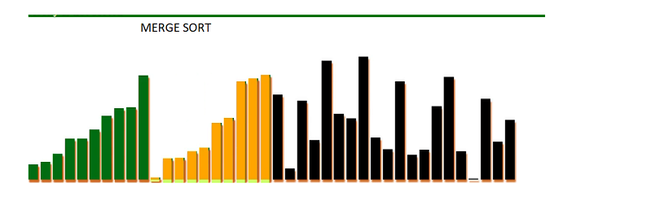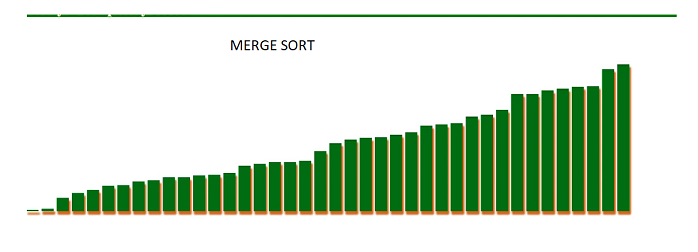# Merge Sort Visualization in JavaScript

• Difficulty Level : Medium
• Last Updated : 25 Aug, 2021

GUI(Graphical User Interface) helps users with better understanding programs. In this article, we will visualize Merge Sort using JavaScript. We will see how the arrays are divided and merged after sorting to get the final sorted array.

Refer:

Approach:

• First, we will generate a random array using the Math. random() function.
• Properties of the canvas are used to make rectangle bars and animations.
• Different colors are used to indicate which arrays are divided and merged.
• The sorting is performed using the JavaScript mergeSort() function.
• The algorithm performs the operation very fast, the timeout() function has been used to slow down the process.

Unsorted list:Sorted list:index.html: Below is the program to visualize the Merge Sort algorithm.

## HTML

 `` `<``html` `lang``=``"en"``>`   `<``head``>` `    ``<``meta` `charset``=``"UTF-8"``>` `    ``<``meta` `name``=``"viewport"` `content="``width``=``device``-width, ` `    ``initial-scale``=``1``.0">    ` ``   `<``body``>` `    ``<``h1` `class``=``"title"` `style` `= ``"color: green; font:lighter; "``>` `        ``Merge Sort Visualizer using JS`   `    ``<``h2` `class``=``"title1"` `style = "background: green; ` `    ``color: white;  font: italic;">Array is not sorted ` `    ``` `  `  `    ``<``canvas` `id``=``"Canvas"``>` `    `  `    ``<``script` `src``=``"mergeSort.js"``>` ``   ``

mergeSort.js: The following is the content for the “mergeSort.js” file used in the above HTML code.

## Javascript

 `// Canvas variables` `var` `canvas, canvaswidth, canvasheight, ctrl;`   `// Call canvasElements() to store height width` `// in above canvas variables` `canvasElements();`   `// 3 array are declared`   `//1) arr is for storing array element ` `//2) itmd for storing intermediate values` `//3) visited is for element which has been sorted` `var` `arr = [], itmd = [], visited = []`     `// Length of unsorted array` `var` `len_of_arr = 40;`   `// Store random value in arr[]` `for` `(``var` `i = 0; i < len_of_arr; i++) {` `    ``arr.push(Math.round(Math.random() * 250) )` `}`   `// Initialize itmd and visited array with 0` `for` `(``var` `i = 0; i < len_of_arr; i++) {` `    ``itmd.push(0)` `    ``visited.push(0)` `}`   `// Merging of two sub array` `// https://www.geeksforgeeks.org/merge-two-sorted-arrays/` `function` `mergeArray(start, end) {` `    ``let mid = parseInt((start + end) >> 1);` `    ``let start1 = start, start2 = mid + 1` `    ``let end1 = mid, end2 = end` `    `  `    ``// Initial index of merged subarray` `    ``let index = start`   `    ``while` `(start1 <= end1 && start2 <= end2) {` `        ``if` `(arr[start1] <= arr[start2]) {` `            ``itmd[index] = arr[start1]` `            ``index = index + 1` `            ``start1 = start1 + 1;` `        ``}` `        ``else` `if``(arr[start1] > arr[start2]) {` `            ``itmd[index] = arr[start2]` `            ``index = index + 1` `            ``start2 = start2 + 1;` `        ``}` `    ``}`   `    ``// Copy the remaining elements of` `    ``// arr[], if there are any` `    ``while` `(start1 <= end1) {` `        ``itmd[index] = arr[start1]` `        ``index = index + 1` `        ``start1 = start1 + 1;` `    ``}`   `    ``while` `(start2 <= end2) {` `        ``itmd[index] = arr[start2]` `        ``index = index + 1` `        ``start2 = start2 + 1;` `    ``}`   `    ``index = start` `    ``while` `(index <= end) {` `        ``arr[index] = itmd[index];` `        ``index++;` `    ``}` `}`   `// Function for showing visualization ` `// effect` `function` `drawBars(start, end) {`   `    ``// Clear previous unsorted bars` `    ``ctrl.clearRect(0, 0, 1000, 1500)`   `    ``// Styling of bars` `    ``for` `(let i = 0; i < len_of_arr; i++) {`   `        ``// Changing styles of bars` `        ``ctrl.fillStyle = ``"black"` `        ``ctrl.shadowOffsetX = 2` `        ``ctrl.shadowColor = ``"chocolate"``;` `        ``ctrl.shadowBlur = 3;` `        ``ctrl.shadowOffsetY =5;` `       `  `        `  `        ``// Size of rectangle of bars` `        ``ctrl.fillRect(25 * i, 300 - arr[i], 20, arr[i])` `        `  `        ``if` `(visited[i]) {` `            ``ctrl.fillStyle = ``"#006d13"` `            ``ctrl.fillRect(25 * i, 300 - arr[i], 20, arr[i])` `            ``ctrl.shadowOffsetX = 2` `        ``}` `    ``}`   `    ``for` `(let i = start; i <= end; i++) {` `        ``ctrl.fillStyle = ``"orange"` `        ``ctrl.fillRect(25 * i, 300 - arr[i], 18, arr[i])` `        ``ctrl.fillStyle = ``"#cdff6c"` `        ``ctrl.fillRect(25 * i,300, 18, arr[i])` `        ``visited[i] = 1` `    ``}` `}`   `// Waiting interval between two bars` `function` `timeout(ms) {` `    ``return` `new` `Promise(resolve => setTimeout(resolve, ms));` `}`     `// Merge Sorting` `const mergeSort = async (start, end) => {` `    ``if` `(start < end) {` `        ``let mid = parseInt((start + end) >> 1)` `        ``await mergeSort(start, mid)` `        ``await mergeSort(mid + 1, end)` `        ``await mergeArray(start, end)` `        ``await drawBars(start, end)`   `        ``// Waiting time is 800ms` `        ``await timeout(800)` `    ``}` `}`   `// canvasElements function for storing` `// width and height in canvas variable` `function` `canvasElements() {` `    ``canvas = document.getElementById(``"Canvas"``)` `    ``canvas.width = canvas.height = 1000` `    ``canvaswidth = canvas.width` `    ``canvasheight = canvas.height` `    ``ctrl = canvas.getContext(``"2d"``)` `}`   `// Asynchronous MergeSort function ` `const performer = async () => {` `    ``await mergeSort(0, len_of_arr - 1)` `    ``await drawBars()`   `    ``// Code for change title1 text ` `    ``const title1_changer = document.querySelector(``".title1"``)` `    ``title1_changer.innerText = ``"Array is completely sorted"` `}` `performer()`

Output:

My Personal Notes arrow_drop_up
Recommended Articles
Page :